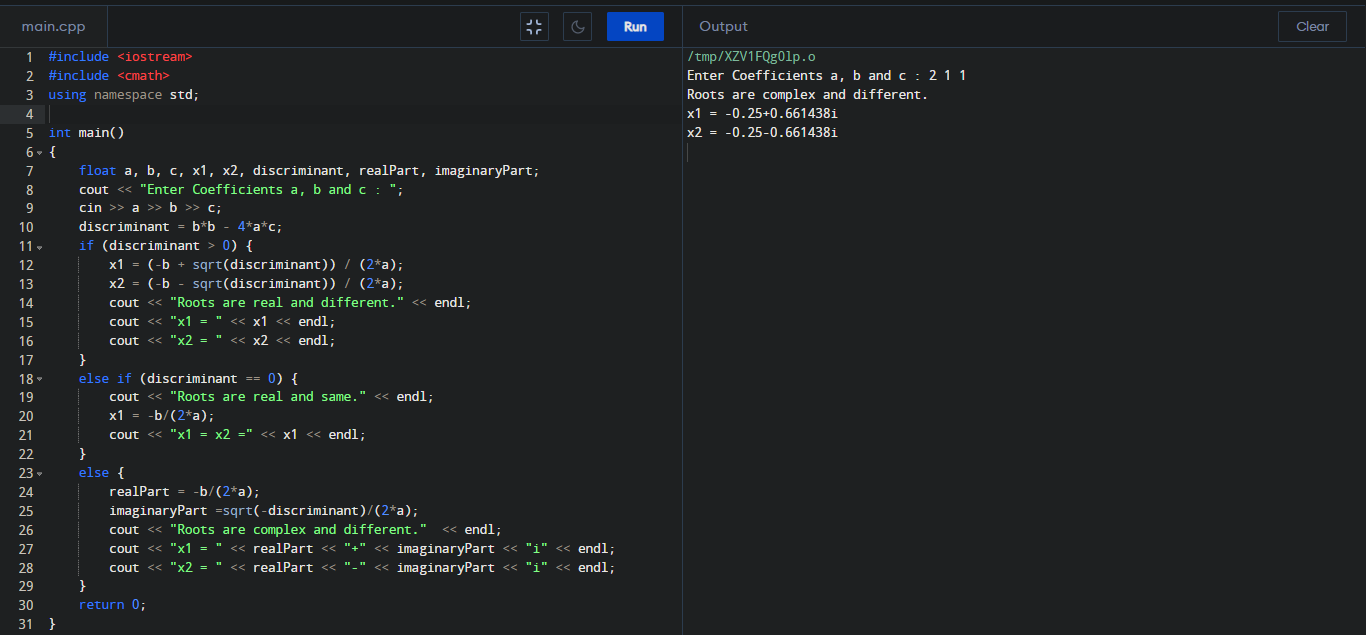C++ Program to find roots of a Quadratic Equation

How can we find roots of a Quadratic equation in C++? We can solve any quadratic equation by completing the square, rewriting part of the equation as a perfect square trinomial. If we complete the square on the generic equation ax2 + bx + c = 0 and then solve for x, then we will get the root.

#include <iostream>
#include <cmath>
using namespace std;

int main()
{
float a, b, c, x1, x2, discriminant, realPart, imaginaryPart;
cout << "Enter Coefficients a, b and c : ";
cin >> a >> b >> c;
discriminant = b*b - 4*a*c;

if (discriminant > 0)
{
x1 = (-b + sqrt(discriminant)) / (2*a);
x2 = (-b - sqrt(discriminant)) / (2*a);
cout << "Roots are real and different." << endl;
cout << "x1 = " << x1 << endl;
cout << "x2 = " << x2 << endl;
}
else if (discriminant == 0)
{
cout << "Roots are real and same." << endl;
x1 = -b/(2*a);
cout << "x1 = x2 =" << x1 << endl;
}
else
{
realPart = -b/(2*a);
imaginaryPart =sqrt(-discriminant)/(2*a);
cout << "Roots are complex and different."  << endl;
cout << "x1 = " << realPart << "+" << imaginaryPart << "i" << endl;
cout << "x2 = " << realPart << "-" << imaginaryPart << "i" << endl;
}

return 0;
}

Output :